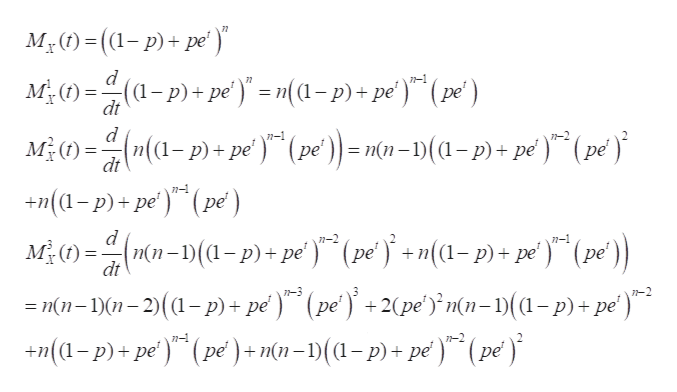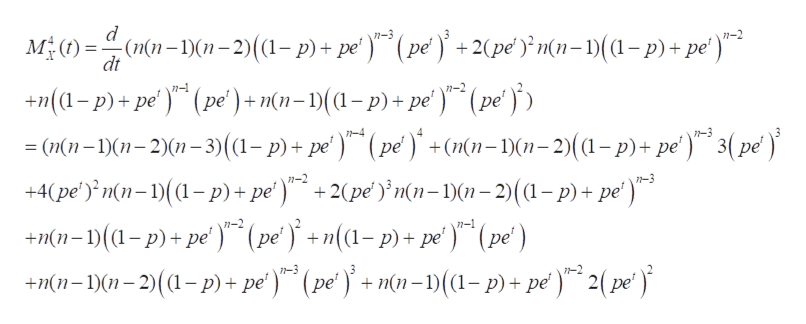# Escalate - Show every mynute steps in DETAILED!

Question
19 views

Escalate - Show every mynute steps in DETAILED!

check_circle

Step 1

Given X is binomial distribution with parameters n,p.
The moment generating funciton of binomial distribution is given below

Step 2

If X is a random variable with with moment generating function MX(t) then
E[Xn] = MXn(0)
where MXn(t) is nth derivative of MX(t).
Deriving the moment generating function three times we get the following
We use the chain rule to derivate the given moment generating function.help_outlineImage TranscriptioncloseMy)=((1-p)+pe (a-p) + pe) - m(a-p) + pe') (pe) M)n(a-p)pe (pe)- mn-(a-p)+ pe ) d 7-1 77 ре dt d (pe п(n dt +(a-p)+pe) (pe) ME)n-(-p) + pe) (pe+n(a-p)+ pe (pe n-1 п(п-1) dt ре ре n-2 7-3 (pe' ) +2(pe'n(n-)(1-p)+pe') =nn-1)-2)(a-p)+ pe) n- 1-2 +(p)+pe) (pe) + m(n- 1)(a-p) + pe)" (pe) fullscreen
Step 3

Derivating the moment generating function for fou...help_outlineImage Transcriptionclosed M )(n-n-2)((1- p)+ pe) (pe' +2(pe )}mn=1)(1-p)+pe') n-3 n-2 dt +n(a-p)+pe' (pe') + mn=1)((1-p)+ pe') (pe = (n(n-1)(1-2)(1-3)(1-p) + pe') (pe)+(nn-1)(1-2)((1-p)+ pe )*° 3( p¢ ) +4(pe') n(n-(p)+ pe')+2(pe'n(n-1)(1-2)((1-p)+ pe') 7-4 1-3 n-2 +n(1-)(a-p)+pe) (pe+n(1-p)+ pe ) (pe' ) +Mn-)01-2)(a-p)+pe) (pe+mn-1)(1-p) + pe) 2(pe) n-1 n-3 fullscreen

### Want to see the full answer?

See Solution

#### Want to see this answer and more?

Solutions are written by subject experts who are available 24/7. Questions are typically answered within 1 hour.*

See Solution
*Response times may vary by subject and question.
Tagged in

### Probability